# Classifying Numbers Natural Numbers Whole Numbers Integers Rational

• Slides: 26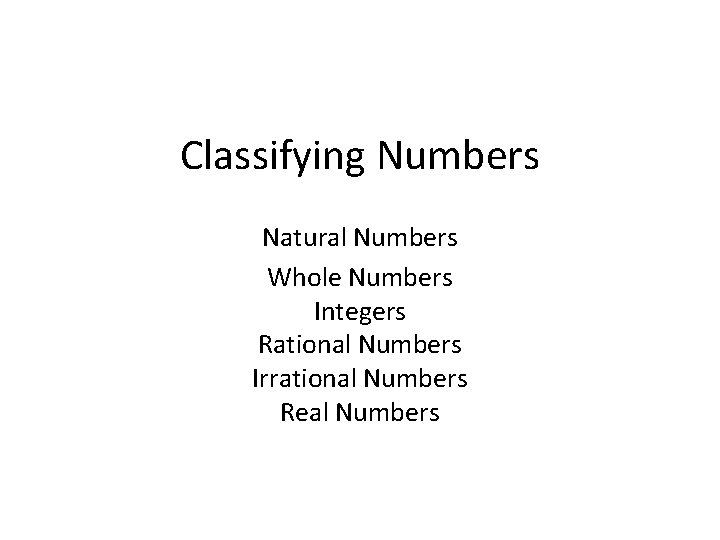Classifying Numbers Natural Numbers Whole Numbers Integers Rational Numbers Irrational Numbers Real Numbers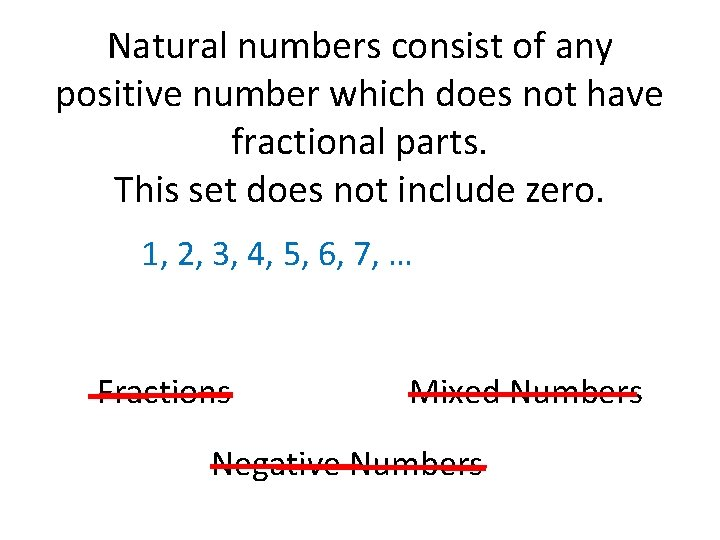Natural numbers consist of any positive number which does not have fractional parts. This set does not include zero. 1, 2, 3, 4, 5, 6, 7, … Fractions Mixed Numbers Negative Numbers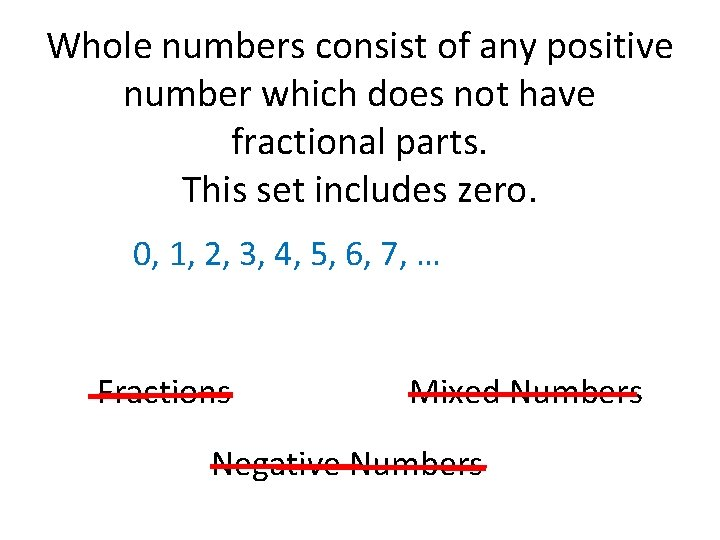Whole numbers consist of any positive number which does not have fractional parts. This set includes zero. 0, 1, 2, 3, 4, 5, 6, 7, … Fractions Mixed Numbers Negative Numbers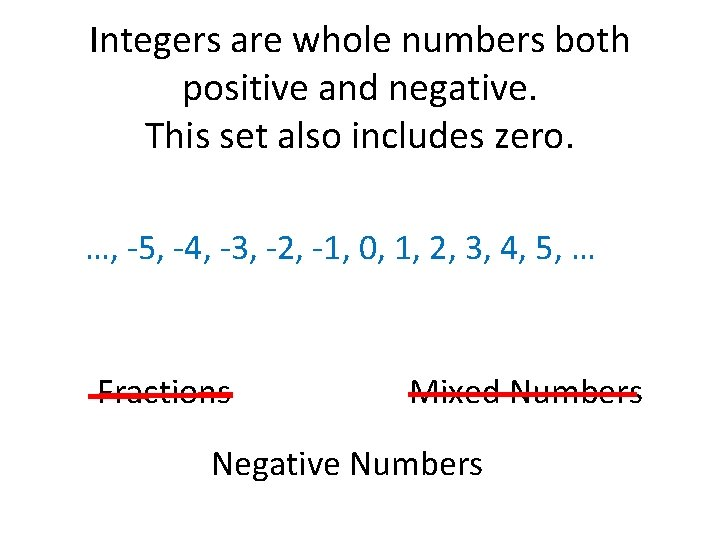Integers are whole numbers both positive and negative. This set also includes zero. …, -5, -4, -3, -2, -1, 0, 1, 2, 3, 4, 5, … Fractions Mixed Numbers Negative Numbers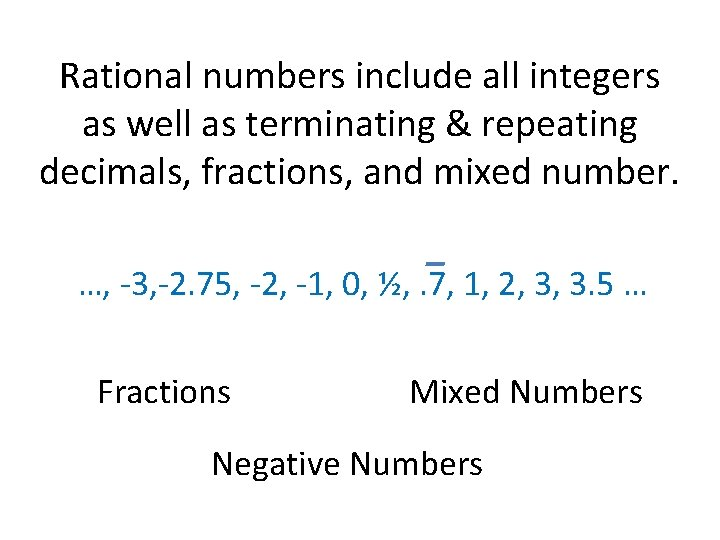Rational numbers include all integers as well as terminating & repeating decimals, fractions, and mixed number. …, -3, -2. 75, -2, -1, 0, ½, . 7, 1, 2, 3, 3. 5 … Fractions Mixed Numbers Negative Numbers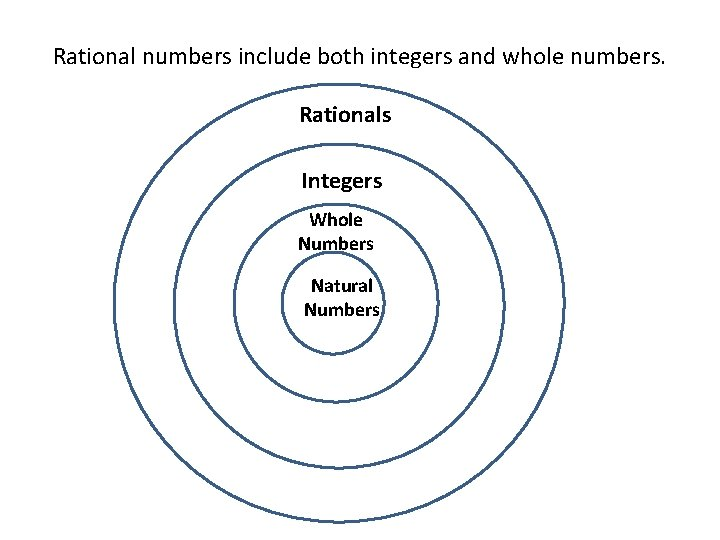Rational numbers include both integers and whole numbers. Rationals Integers Whole Numbers Natural Numbers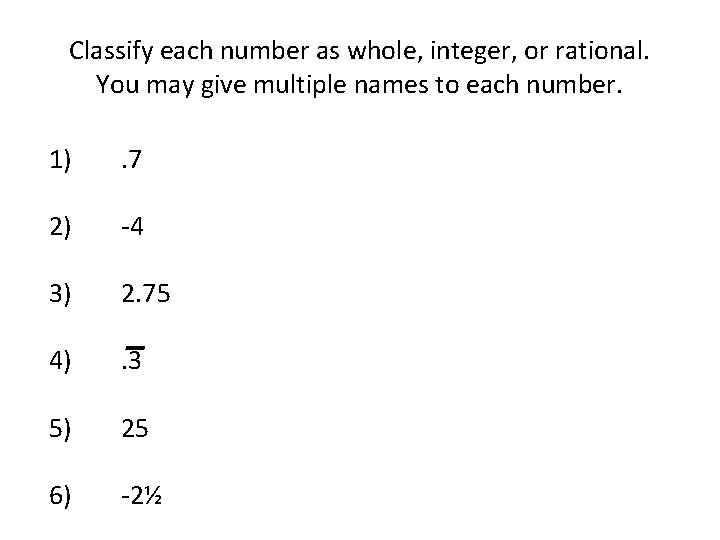Classify each number as whole, integer, or rational. You may give multiple names to each number. 1) . 7 2) -4 3) 2. 75 4) . 3 5) 25 6) -2½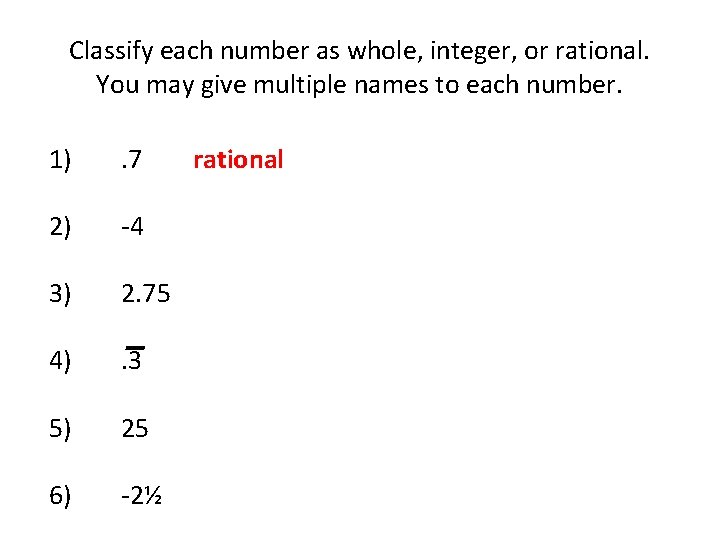Classify each number as whole, integer, or rational. You may give multiple names to each number. 1) . 7 2) -4 3) 2. 75 4) . 3 5) 25 6) -2½ rational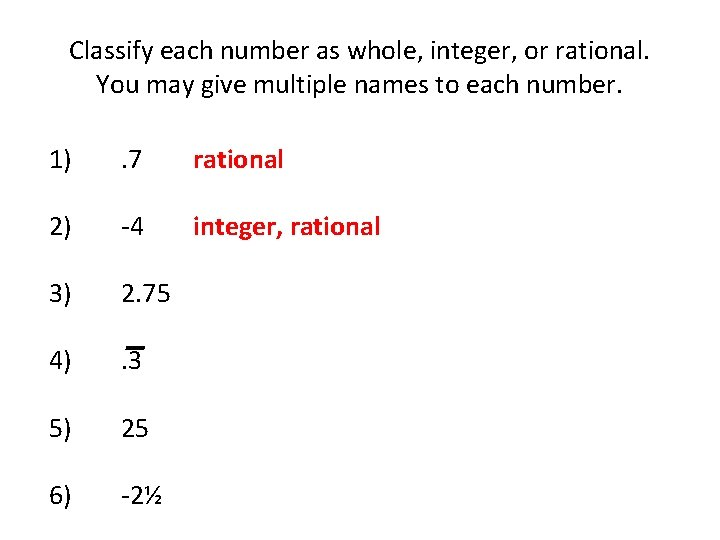Classify each number as whole, integer, or rational. You may give multiple names to each number. 1) . 7 rational 2) -4 integer, rational 3) 2. 75 4) . 3 5) 25 6) -2½Classify each number as whole, integer, or rational. You may give multiple names to each number. 1) . 7 rational 2) -4 integer, rational 3) 2. 75 rational 4) . 3 5) 25 6) -2½Classify each number as whole, integer, or rational. You may give multiple names to each number. 1) . 7 rational 2) -4 integer, rational 3) 2. 75 rational 4) . 3 5) 25 6) -2½ rationalClassify each number as whole, integer, or rational. You may give multiple names to each number. 1) . 7 rational 2) -4 integer, rational 3) 2. 75 rational 4) . 3 rational 5) 25 whole, integer, rational 6) -2½Classify each number as whole, integer, or rational. You may give multiple names to each number. 1) . 7 rational 2) -4 integer, rational 3) 2. 75 rational 4) . 3 rational 5) 25 whole, integer, rational 6) -2½ rationalWhat isn’t a rational number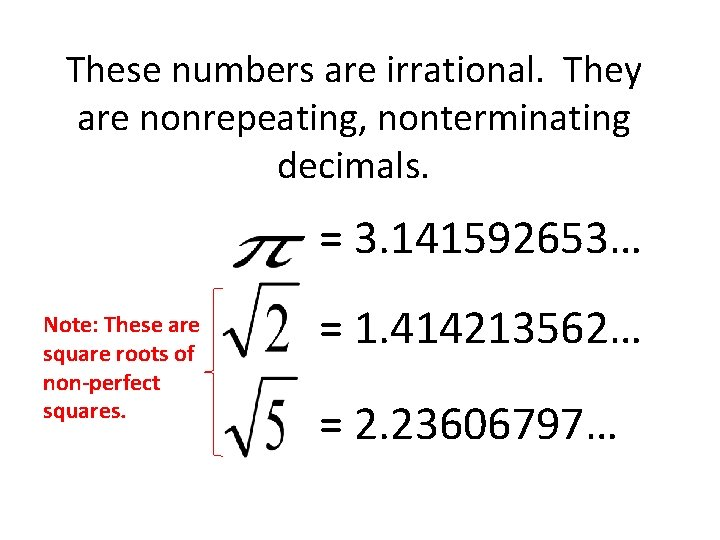These numbers are irrational. They are nonrepeating, nonterminating decimals. = 3. 141592653… Note: These are square roots of non-perfect squares. = 1. 414213562… = 2. 23606797…The set of rational numbers and irrational numbers comprise the set of real numbers. Rationals Integers Whole Natural Real Numbers IrrationalsDecide whether each number is rational or irrational. 1) 2) 3) -6 4) 5) 2. 454545… 6) 7. 25 7) 8)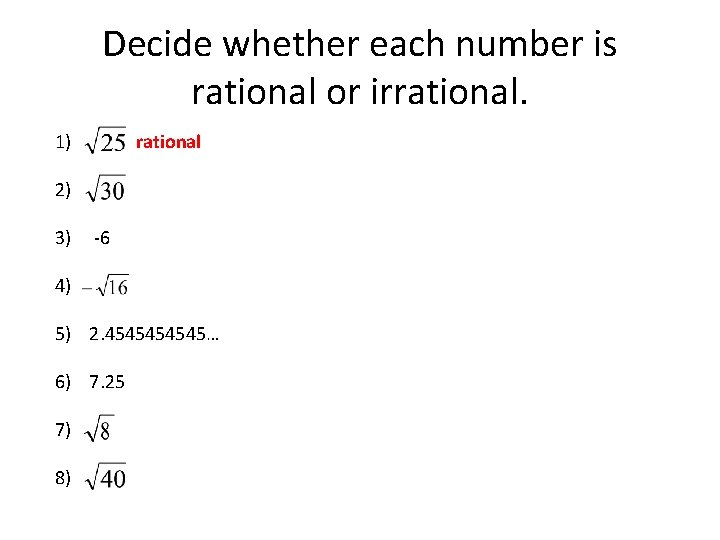Decide whether each number is rational or irrational. 1) rational 2) 3) -6 4) 5) 2. 454545… 6) 7. 25 7) 8)Decide whether each number is rational or irrational. 1) rational 2) irrational 3) -6 4) 5) 2. 454545… 6) 7. 25 7) 8)Decide whether each number is rational or irrational. 1) rational 2) irrational 3) -6 rational 4) 5) 2. 454545… 6) 7. 25 7) 8)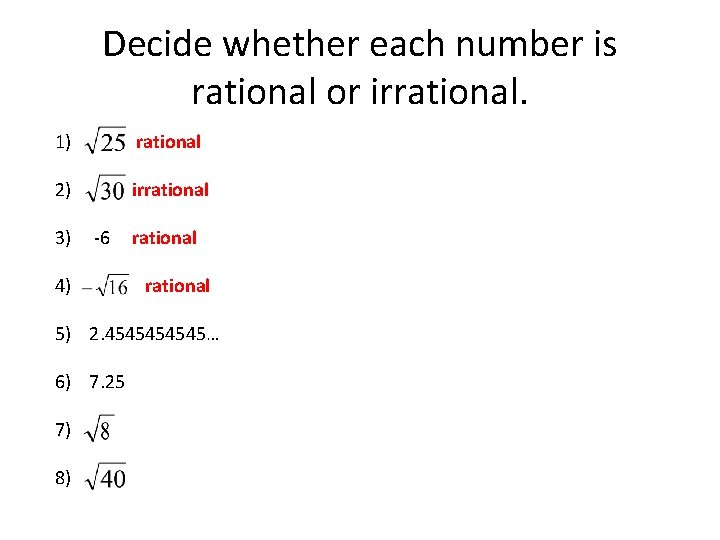Decide whether each number is rational or irrational. 1) rational 2) irrational 3) -6 4) rational 5) 2. 454545… 6) 7. 25 7) 8)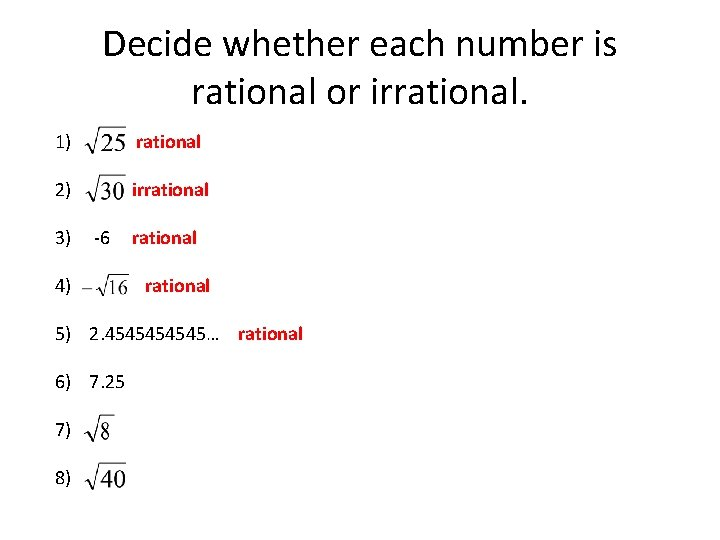Decide whether each number is rational or irrational. 1) rational 2) irrational 3) -6 4) rational 5) 2. 454545… rational 6) 7. 25 7) 8)Decide whether each number is rational or irrational. 1) rational 2) irrational 3) 4) -6 rational 5) 2. 454545… rational 6) 7. 25 rational 7) 8)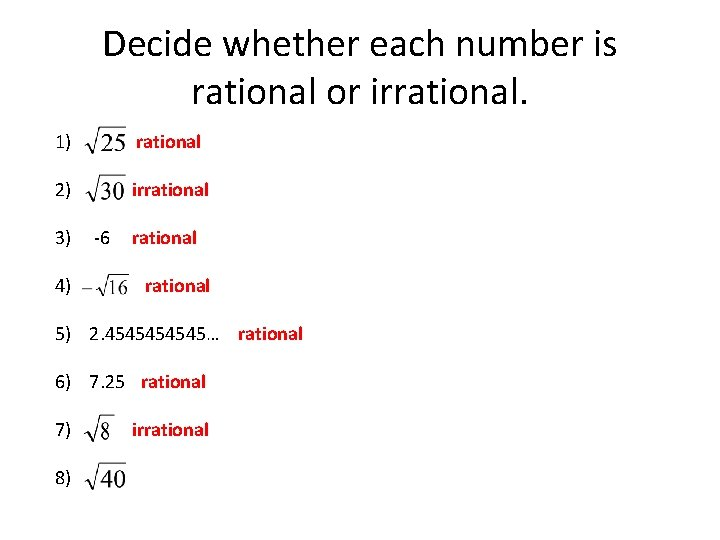Decide whether each number is rational or irrational. 1) rational 2) irrational 3) 4) -6 rational 5) 2. 454545… rational 6) 7. 25 rational 7) 8) irrational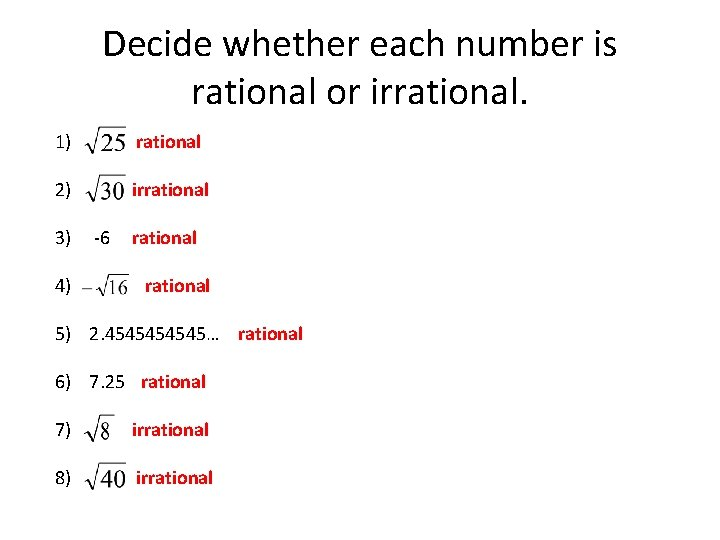Decide whether each number is rational or irrational. 1) rational 2) irrational 3) 4) -6 rational 5) 2. 454545… rational 6) 7. 25 rational 7) irrational 8) irrationalRationals Integers Whole Natural Real Numbers Irrationals Numbers that are NOT real.